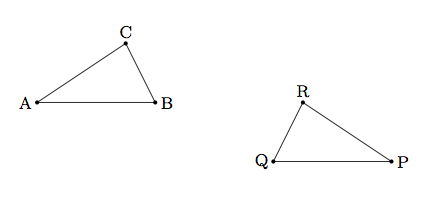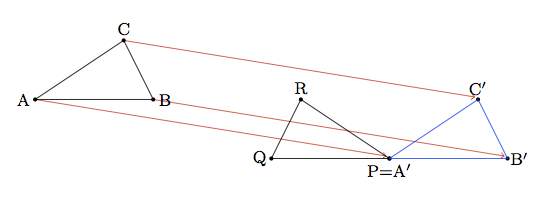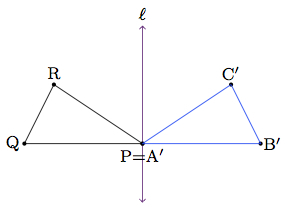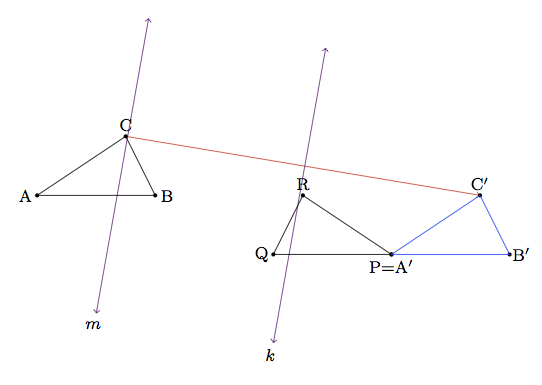# Showing a triangle congruence: a particular case

Alignments to Content Standards: G-CO.A.5

Triangles $ABC$ and $PQR$ pictured below are congruent:1. Show the congruence using rigid motions of the plane.
2. Can the congruence be shown with a single translation, rotation, or reflection? Explain.
3. Is it possible to show the congruence using only translations? Explain.
4. Is it possible to show the congruence using only rotations? Explain.
5. Is it possible to show the congruence using only reflections? Explain.

## IM Commentary

This task provides experience working with transformations of the plane and also an abstract component analyzing the effects of the different transformations. If computer software is available, this would allow students to experiment quickly with the different transformations and help them visualize the answers to (b) through (e). They can also do this by hand but ample time will need to be set aside for the task.

Regarding parts (c) through (e) we can determine visually if translations alone suffice to show a congruence because a composition of translations is a translation and so the triangle will be displaced but not altered otherwise. For rotations it is much harder to visualize the possibilities and it is even more difficult to visualize what happens when we compose rotations so much experimentation is in order here. Finally reflections are unique in that compositions of reflections produce all possible rigid motions of the plane. In fact, only one, two, or three reflections is required to produce any rigid motion.

The task is well suited for group work. This can be particularly effective for part (a) where the groups can be encouraged to be creative in their choice of transformations. Sharing and understanding many different constructions for part (a) should help complete the other parts of the task.

Several of the standards for mathematical practice are illustrated by this task:

• MP2, Reason Abstractly and Quantitatively: this is particularly relevant for part (b) where students must find some property of the translations and rotations that shows that they can not be used alone to show the triangle congruence.
• MP3, Construct Viable Arguments and Critique the Reasoning of Others: this task is ideally suited for group work since there are multiple solutions for part (a) and both (b) and (c) are relatively open ended.
• MP5, Use Appropriate Tools Strategically: this is an ideal task for computer graphing technology.

## Solution

1. One way to show the congruence is to first translate vertex $A$ to $P$ which is shown below:The image of $\triangle ABC$ after this translation is denoted $\triangle A^\prime B^\prime C^\prime$. A reflection about the indicated line $\ell$ then completes the congruence between $\triangle ABC$ and $\triangle PQR$:This last step assumes that $Q$, $P$, and $B^\prime$ are collinear: they appear to be collinear and in the absence of coordinates we assume that this is the case.

2. The congruence can not be established with a single translation, rotation, or reflection. Neither a translation nor a rotation can work because the vertices of $\triangle ABC$ are listed in counterclockwise order while those of $\triangle PQR$ are listed in clockwise order. This means that $\triangle ABC$ and $\triangle PQR$ have opposite orientations. But translations and rotations do not change the orientation and so neither a translation nor a rotation can establish the congruence.

The argument of the previous paragraph does not apply to reflections because they do change the orientation. Therefore a single reflection could, in principle, work to show that $\triangle ABC$ is congruent to $\triangle PQR$. In order to see that this is not the case, we draw the line segments connecting corresponding vertices of the two triangles:If a single reflection were to map $\triangle ABC$ to $\triangle PQR$ then the line $\ell$ of the reflection would be the perpendicular bisector of all three red segments. This is not possible and so the congruence can not be shown with a single reflection.

3. We saw in part (b) that translations preserve orientation and so no composition of translations will be sufficient to show the congruence between $\triangle ABC$ and $\triangle PQR$ (since they have opposite orientations). Alternatively, if there were such a translation, it would send $A$ to $P$, $Q$, or $R$ and we can check by hand that none of these work.

4. As in part (c), since rotations preserve orientation, no composition of rotations will show the congruence between $\triangle ABC$ and $\triangle PQR$.

5. Reflections are sufficient to exhibit this triangle congruence or any other congruence. In this case, for part (a) we used a translation followed by a reflection to exhibit the congruence. So if we can rewrite the translation as a composition of reflections then we will have the entire congruence done by a sequence of reflections.

The key to realizing a translation as a composition of reflections is to use a pair of lines which are parallel to one another and perpendicular to the direction of translation. Specifically, suppose we let $m$ be the perpendicular to $\overleftrightarrow{CC^\prime}$ through $C$ and $k$ the perpendicular bisector of $\overline{CC^\prime}$ as in the picture below:Reflecting $\triangle ABC$ about $m$ and then about $k$ maps $\triangle ABC$ to $\triangle A^\prime B^\prime C^\prime$. One final reflection about $\ell$ completes the congruence of $\triangle ABC$ with $\triangle PQR$ using three reflections. We already saw that one reflection is never sufficient and two reflections will not work either (because reversing the orientation twice is the same as preserving the original orientation). So three reflections are necessary to show the congruence.